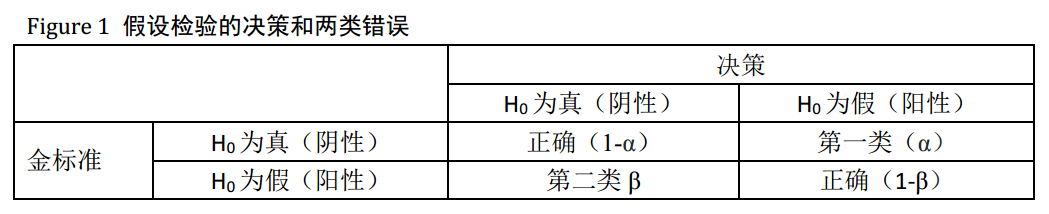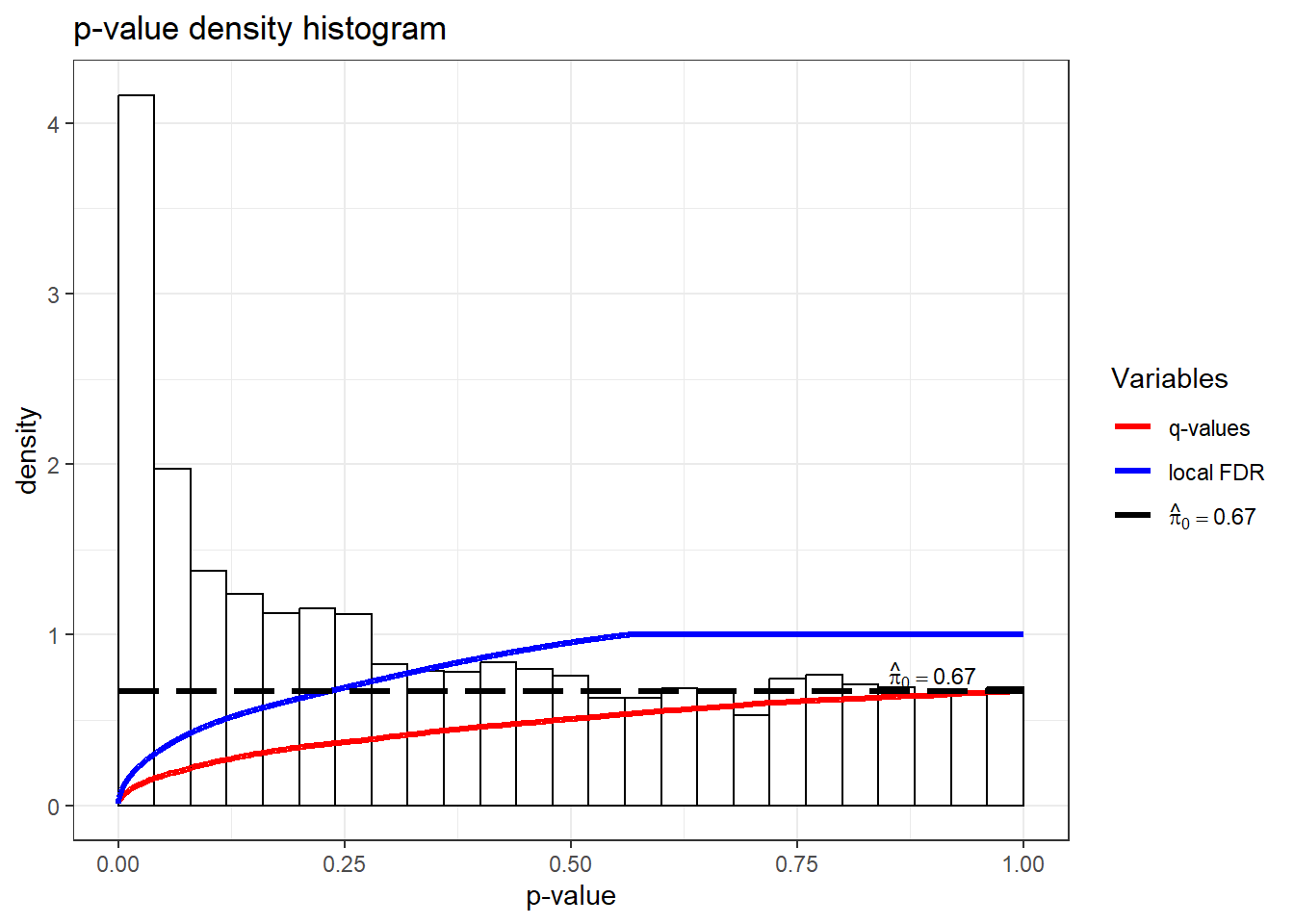# 假设检验# 假阳性和假阴性

假阳性意思为，看起来显著但是实际上是阴性的结果，在实验中如果选择了假阳性的结果会导致后面的实验失败。

假阴性意思为，看起来是不显著但是实际上是阳性的结果，在实验中如果假阴性检出率过大，会影响新的独特的实验结果，以及对表达差异不大的基因会漏样，进而掩盖那些真正有差异的基因。

# 检验的势

检验的势(power of the test)定义为在原假设是错误的情况下正确拒绝原假设的概率。检验的势等于1减去犯第二类错误的概率：1-β；当两个总体参数的确存在差异时，所使用的统计检验能够发现这种差异的概率。 1-β最好大于80%。

$Z_\beta = \frac{\sqrt{n}\delta}{\sigma}-Z_\alpha$ 其中 n为样本量；$$\delta$$偏差；$$\sigma$$总体标准差；$$Z_\alpha$$:标准正态分布临界值，单侧检验取单侧，双侧取双侧;$$Z_\beta$$:标准正态分布上侧尾部$$\beta$$临界值。

# P值的矫正方法

## FDR计算

FDR错误发现率，假阳性/真阳性+假阳性，让其小于0.05，这样允许存在假阳性的结果，是一种折中的方法；例如20000基因，其中1000个是差异基因，其中假阳性基因数目小于50个，可以接受。

library(qvalue)
#hedenfalk  P-values and test-statistics from the Hedenfalk et al. (2001) gene expression dataset
data("hedenfalk")
pvalue <- hedenfalk$p qobj <- qvalue(pvalue) # q值 qvalue <- qobj$qvalues
summary(qobj)
##
## Call:
## qvalue(p = pvalue)
##
## pi0: 0.669926
##
## Cumulative number of significant calls:
##
##           <1e-04 <0.001 <0.01 <0.025 <0.05 <0.1   <1
## p-value       15     76   265    424   605  868 3170
## q-value        0      0     1     73   162  319 3170
## local FDR      0      0     3     30    85  167 2241
hist(qobj)π0:the proportion of true null hypotheses π0,

# Ref

1. 概率论与数理统计. 作者：— 茆诗松, 周纪芗编著. 索书号：O21-43/4434. ISBN： 978-7-5037-5332-9. 出版社：中国统计出版社2007↩︎

2. Storey JD, Bass AJ, Dabney A, Robinson D (2020). qvalue: Q-value estimation for false discovery rate control. R package version 2.22.0, http://github.com/jdstorey/qvalue.↩︎